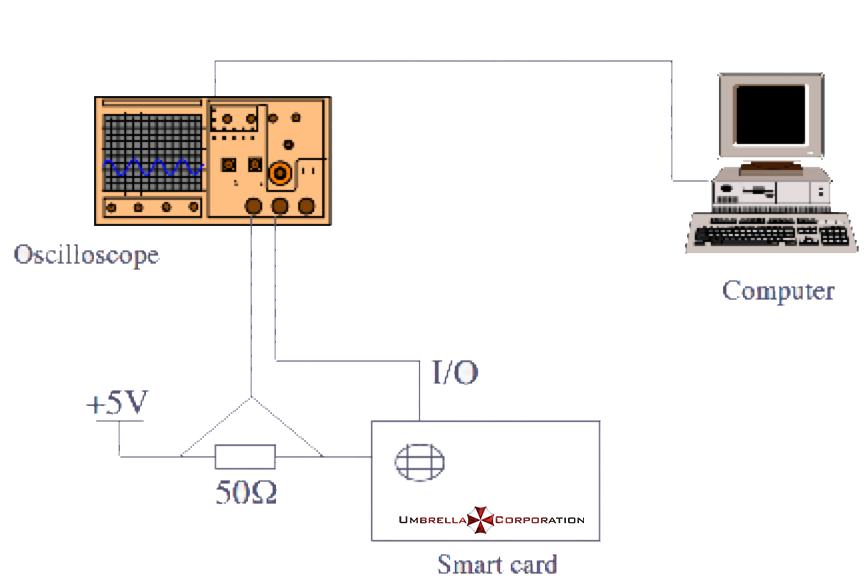# RSA 侧信道攻击¶

## 例子¶014b05e1a09668c83e13fda8be28d148568a2342aed833e0ad646bd45461da2decf9d538c2d3ab245b272873beb112586bb7b17dc4b30f0c5408d8b03cfbc8388b2bd579fb419a1cac38798da1c3da75dc9a74a90d98c8f986fd8ab8b2dc539768beb339cadc13383c62b5223a50e050cb9c6b759072962c2b2cf21b4421ca73394d9e12cfbc958fc5f6b596da368923121e55a3c6a7b12fdca127ecc0e8470463f6e04f27cd4bb3de30555b6c701f524c8c032fa51d719901e7c75cc72764ac00976ac6427a1f483779f61cee455ed319ee9071abefae4473e7c637760b4b3131f25e5eb9950dd9d37666e129640c82a4b01b8bdc1a78b007f8ec71e7bad48046


### 分析¶

1. b 为偶数时，$a^b \bmod c = ({a^2}^{b/2}) \bmod c$
2. b 为奇数时，$a^b \bmod c = ({a^2}^{b/2} \times a) \bmod c$

int PowerMod(int a, int b, int c)
{
int ans = 1;
a = a % c;
while(b>0) {
if(b % 2 == 1) // 当b为奇数时会多执行下面的指令
ans = (ans * a) % c;
b = b/2;
a = (a * a) % c;
}
return ans;
}f = open('./data.txt')
print('point number:', len(data))

start_point = 225   # 开始分析的点
mid = 50            # 采样点间隔
fence = 228         # 高低电平分界线

bin_array = []

for point_index in range(start_point, len(data), mid):
if float(data[point_index]) > fence:
bin_array.append(1)
else:
bin_array.append(0)

bin_array2 = []
flag1 = 0
flag2 = 0
for x in bin_array:
if x:
if flag1:
flag2 = 1
else:
flag1 = 1
else:
if flag2:
bin_array2.append(1)
else:
bin_array2.append(0)
flag1 = 0
flag2 = 0

# d_bin = bin_array2[::-1]
d_bin = bin_array2
d = "".join(str(x) for x in d_bin)[::-1]
print(d)
d_int = int(d,2)
print(d_int)


## 参考资料¶

1. Mangard, S., Oswald, E., Popp, T., 冯登国, 周永彬, & 刘继业. (2010). 能量分析攻击.